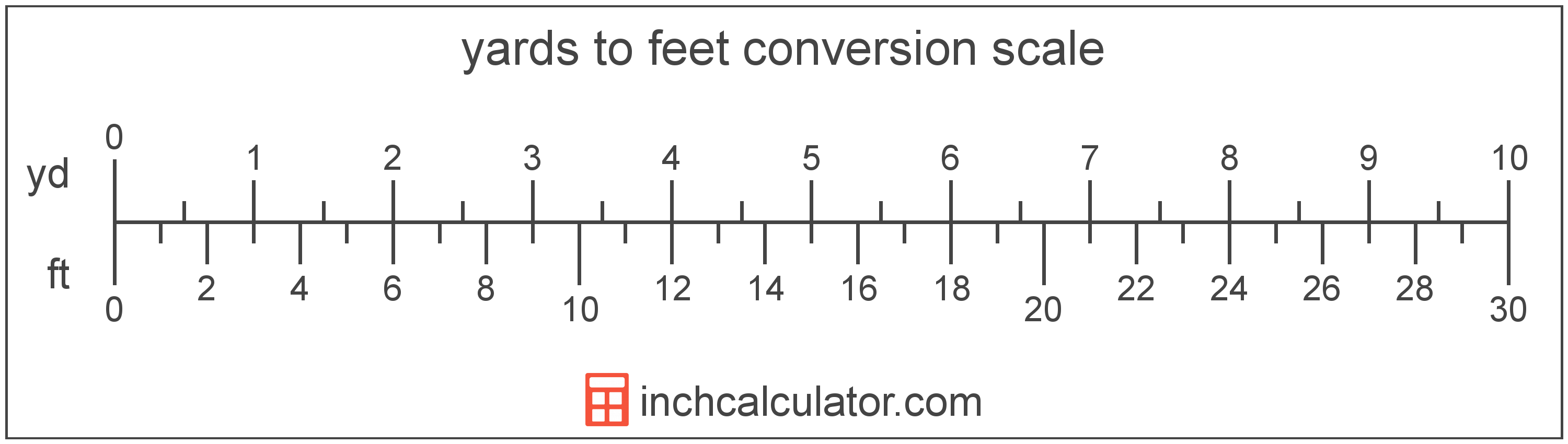# Yards to Feet & Inches Conversion

Enter the length in yards below to get the value converted to feet.

yd
ft
Results in Feet:1 yd = 3 ft
1 yd = 3'
Do you want to convert feet to yards?

## How to Convert Yards to FeetTo convert a yard measurement to a foot measurement, multiply the length by the conversion ratio.

Since one yard is equal to 3 feet, you can use this simple formula to convert:

feet = yards × 3

The length in feet is equal to the yards multiplied by 3.

For example, here's how to convert 5 yards to feet using the formula above.
5 yd = (5 × 3) = 15'

### How Many Feet are in a Yard?

There are 3 feet in a yard, which is why we use this value in the formula above.

1 yd = 3'

Our inch fraction calculator can add yards and feet together, and it also automatically converts the results to US customary, imperial, and SI metric values.

## Yards

The yard is a unit of length measurement equal to 3 feet or 36 inches. The international yard is legally defined to be equal to exactly 0.9144 meters.

The yard is a US customary and imperial unit of length. Yards can be abbreviated as yd; for example, 1 yard can be written as 1 yd.

## Feet

The foot is a unit of linear length measure equal to 12 inches or 1/3 of a yard. Because the international yard is legally defined to be equal to exactly 0.9144 meters, one foot is equal to 0.3048 meters.

The foot is a US customary and imperial unit of length. Feet can be abbreviated as ft; for example, 1 foot can be written as 1 ft.

Feet can also be denoted using the symbol, otherwise known as a prime, though a single-quote (') is often used instead of the prime symbol for convenience. Using the prime symbol, 1 ft can be written as 1′.

The foot is most commonly measured using either a standard 12" ruler or a tape measure, though there are many other measuring devices available. Feet are sometimes referred to as linear feet, which are simply the measurement of length in feet.

You might be interested in our feet and inches calculator, which can add feet with inches, centimeters, or meters.

We recommend using a ruler or tape measure for measuring length, which can be found at a local retailer or home center. Rulers are available in imperial, metric, or combination with both values, so make sure you get the correct type for your needs.

Need a ruler? Try our free downloadable and printable rulers, which include both imperial and metric measurements.

## Yard to Foot Conversion Table

Yard measurements converted to feet
Yards Feet
1 yd 3'
2 yd 6'
3 yd 9'
4 yd 12'
5 yd 15'
6 yd 18'
7 yd 21'
8 yd 24'
9 yd 27'
10 yd 30'
11 yd 33'
12 yd 36'
13 yd 39'
14 yd 42'
15 yd 45'
16 yd 48'
17 yd 51'
18 yd 54'
19 yd 57'
20 yd 60'
21 yd 63'
22 yd 66'
23 yd 69'
24 yd 72'
25 yd 75'
26 yd 78'
27 yd 81'
28 yd 84'
29 yd 87'
30 yd 90'
31 yd 93'
32 yd 96'
33 yd 99'
34 yd 102'
35 yd 105'
36 yd 108'
37 yd 111'
38 yd 114'
39 yd 117'
40 yd 120'

## References

1. National Bureau of Standards, Refinement of Values for the Yard and the Pound, https://www.ngs.noaa.gov/PUBS_LIB/FedRegister/FRdoc59-5442.pdf
2. National Institute of Standards and Technology, U.S. Survey Foot: Revised Unit Conversion Factors, https://www.nist.gov/pml/us-surveyfoot/revised-unit-conversion-factors